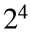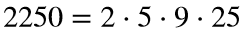# Problem 58018. List the Fermi-Dirac primes

The Fermi-Dirac “primes” are prime powers with exponents that are powers of 2. The first nine terms of the sequence are 2, 3, 4, 5, 7, 9, 11, 13, and 16. Six of the terms are primes; two are squares of primes; and the remaining term, 16, is.
The name comes from an analogy with particle physics because every number can be written as the product of a unique subset of the Fermi-Dirac primes. For example,.
Write a function analogous to primes(n) that lists the Fermi-Dirac primes less than or equal to n.

### Solution Stats

66.67% Correct | 33.33% Incorrect
Last Solution submitted on Oct 17, 2023

### Community Treasure Hunt

Find the treasures in MATLAB Central and discover how the community can help you!

Start Hunting!